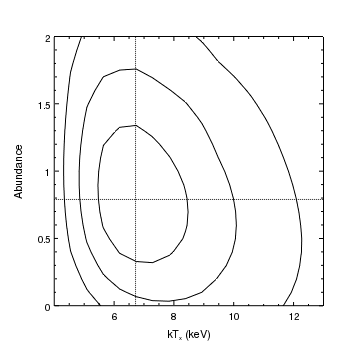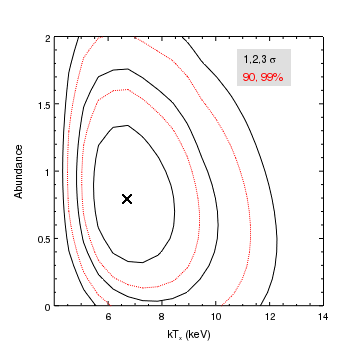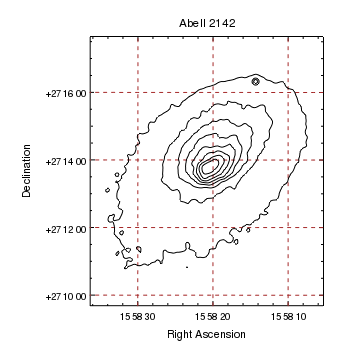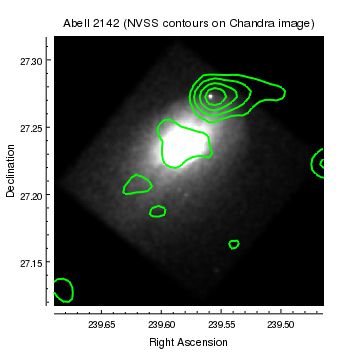Gallery: Contours

1) A simple contor plot

Contour plots are used to draw contours of 2D images which can be read from a FITS file, a two-dimensional array, or given as a set of (x, y, z) points. In this example the data is read from a file, where the image represents the statistic values from a fit of a thermal plasma model to a galaxy cluster spectrum (i.e. the results of a region-projection call in Sherpa).Version: Postscript; PDF
add_contour("contours.fits",[53.6454,57.5304,63.1794],["wcs","params"])
set_data_aspect_ratio("")

limits(X_AXIS,4,13)
limits(Y_AXIS,0,2)

set_plot_xlabel("kT_x (keV)")
set_plot_ylabel("Abundance")


The add_contour call is used to create the contour plot; in this example we give it the file name, an array of contour values, and tell it to use the coordinate information in the image to label the axes.

If the contour levels were not given then ChIPS would have created a set of levels based on the data range of the image; the levels used are accessible via the levels field of the object returned by get_contour, as shown below. For more information see the Controlling the contour levels section of the "Introduction to contours" thread.

The image being displayed contains coordinate information to map from pixel location to "physical" space; in this case the X axis refers to the temperature of a Mekal plasma model (kTx) and the Y axis the abundance of the model, as shown by the dmlist output:

chips> !dmlist contours.fits cols

--------------------------------------------------------------------------------
Columns for Image Block cont.dat_IMAGE
--------------------------------------------------------------------------------

ColNo  Name                 Unit        Type             Range
1   cont.dat_IMAGE[21,21]              Real8(21x21)   -Inf:+Inf

--------------------------------------------------------------------------------
Physical Axis Transforms for Image Block cont.dat_IMAGE
--------------------------------------------------------------------------------

Group# Axis#
1   1,2    params(kTx      ) = (+3.7250) +(+0.550)* ((#1)-(+0.50))
(Abundance)   (-0.050 )  (+0.10 )  ((#2) (+0.50))

--------------------------------------------------------------------------------
World Coordinate Axis Transforms for Image Block cont.dat_IMAGE
--------------------------------------------------------------------------------

Group# Axis#


If the plot had been created by saying

chips> add_contour("contours.fits", [53.6454,57.5304,63.1794])


then the axes would have been labelled using logical coordinates, going from 1 to 21 on both axes in this case.

The add_vline and add_hline calls create a vertical and horizontal line respectively; these lines will extend to the edge of the plot whatever limits are chosen for the axes. Note that the coordinate values used in these calls refer to the params coordinate system, so the call limits(X_AXIS, 4, 13) refers to 4 to 13 keV in this case.

The preferences for contours can be found by using the get_preference call:

chips> print (get_preference("contour"))
contour.stem           : ctr
contour.color          : default
contour.thickness      : 1
contour.levels         : []
contour.numlevels      : 5
contour.style          : solid
contour.algorithm      : marching
contour.mode           : nice
contour.interval       : 10
contour.depth          : default



The settings for the current contour can be found by using the get_contour routine:

chips> get_contour()

algorithm = 1
color = default
depth = 100
id = None
interval = 10.0
levels = [53.645400000000002, 57.5304, 63.179400000000001]
mode = arbitrary
numlevels = 5
stem = None
style = solid
thickness = 1.0



2) Two sets of contours in the same plot

In this example, two contours are added to the same plot. The same image is used, since the contours are used to identify different sets of levels (the solid contours represent the one, two, and three-sigma levels whereas the red dotted contours are the 90 and 99 percent confidence limits), but the images could have been different.Version: Postscript; PDF
add_contour("contours.fits",[53.6454,57.5304,63.1794],["wcs","params"])

set_data_aspect_ratio("")
limits(4,14,0,2)

set_label("all",["size",14])

# Place a region behind the labels to highlight them
xr = [10.8,12.8,12.8,10.8]
yr = [1.63,1.63,1.91,1.91]

set_plot_xlabel("kT_x (keV)")
set_plot_ylabel("Abundance")


As with the first example, the levels are explicitly given and the "params" coordinate conversion is used to label the axes.

Two labels are created, and then they are both changed to use a size of 14 by setting the label currency to all. In order to make the labels stand out a filled region is added to the plot, with a depth of 50 so that it appears behind the labels (since the default depth of items is 100).

The info and info_current routines can be used to find out what ChIPS objects are in use:

chips> info()

Window [win1]
Frame [frm1]
Plot [plot1]   (0.15,0.15)  .. (0.90,0.90)
Border bottom [bx1]  top [bx2]  left [by1]  right [by2]
Contour [ctr1]
X Axis [ax1]
Y Axis [ay1]
Contour [ctr2]
Point [pnt1]
Label [lbl1]
Label [lbl2]
Region [reg1]

chips> info_current()

Window [win1]
Frame [frm1]
Plot [plot1]
X Axis [ax1]
Y Axis [ay1]
Contour [ctr2]
Point [pnt1]
Label [lbl1]
Label [lbl2]
Region [reg1]
Coord Sys [Data]
Coord Sys ID [ds0.0.0.3]



WCS projection support

In CIAO 4.11, WCS support in ChIPS is only available for tangent-plane projections (e.g. images with RA--TAN and DEC-TAN images). Other projections - such as the SIN projection - will be displayed in physical, or logical coordinates. For the gallery plots, SIN projection images (such as provided by NVSS) have been converted to TAN projection - using a combination of the remap program from WCSTools and the Montage package.

3) Contouring an image using the WCS information

This example shows how a contour can be displayed using Right Ascension and Declination rather than "sky" or image coordinates.Version: Postscript; PDF
add_contour("a2142_smoothed.fits",[4,11,18,25,32,39,46,53])

set_xaxis(["tickformat","ra2"])
set_yaxis(["tickformat","dec2"])

set_plot_title("Abell 2142")
set_plot_xlabel("Right Ascension")
set_plot_ylabel("Declination")
set_plot(["leftmargin",0.25])
set_yaxis(["offset.perpendicular",75])

set_axis(["majorgrid.visible",True,"majorgrid.color","brown"])
zoom(0.75)


Since the image contains WCS information, as shown by the dmlist call below, the axes are automatically labelled using this coordinate system. To get sexagesimal format for the axis labels, we change the tickformat attribute of the axes to the values "ra" and "dec".

chips> !dmlist a2142_smoothed.fits cols

--------------------------------------------------------------------------------
Columns for Image Block CONVOLVE
--------------------------------------------------------------------------------

ColNo  Name                 Unit        Type             Range
1   CONVOLVE[366,366]                 Real4(366x366) -Inf:+Inf

--------------------------------------------------------------------------------
Physical Axis Transforms for Image Block CONVOLVE
--------------------------------------------------------------------------------

Group# Axis#
1   1,2    sky(x) = (+3109.50) +(+4.0)* ((#1)-(+0.50))
(y)   (+2939.50)  (+4.0)  ((#2) (+0.50))

--------------------------------------------------------------------------------
World Coordinate Axis Transforms for Image Block CONVOLVE
--------------------------------------------------------------------------------

Group# Axis#
1   1,2    EQPOS(RA ) = (+239.5378)[deg] +TAN[(-0.000136667)* (sky(x)-(+4096.50))]
(DEC)   (+27.2755 )           (+0.000136667)  (   (y) (+4096.50))


Although the axes are labelled in sexagesimal format, only decimal format values can be used to specify a position: e.g. to change the axis range or to position a line or label. The get_plot_xrange and get_plot_yrange() routines can be useful to find out the current axis ranges; in this case the output is

chips> get_plot_xrange()
[239.63871920542988, 239.53475475104338]
chips> get_plot_yrange()
[27.178024922552869, 27.277658788728278]


As the data aspect ratio has been set by the add_contour call: e.g.

chips> get_data_aspect_ratio()
'1:1'


then the plot limits are also changed, to maintain this ratio, when the plot area is changed by the call to set_plot.

Since the source is at a moderate latitude the grid lines displayed by setting majorgrid.visible to True do not show strong curvature. The zoom routine changes the limits to display slightly more of the field.

WCS projection support

In CIAO 4.11, WCS support in ChIPS is only available for tangent-plane projections (e.g. images with RA--TAN and DEC-TAN images). Other projections - such as the SIN projection - will be displayed in physical, or logical coordinates. For the gallery plots, SIN projection images (such as provided by NVSS) have been converted to TAN projection - using a combination of the remap program from WCSTools and the Montage package.

4) Displaying contours from DS9

Using the add_ds9_contours routine from the CIAO contributed scripts package, we can also display contour files created by ds9, using its Save Contours menu item.

This allows you to display the contours of an image that is not in the tangent-plane projection. Here we use it to overlay contours from the NVSS survey, which uses the SIN projection, on a Chandra image of Abell 2142.Version: Postscript; PDF
# Load in the add_ds9_contours command

# Display the Chandra data
set_image(["threshold",[0, 20]])

# Overlay the DS9 contours (which should have been
# saved using the 'Save Contours' menu item in the FK5
# coordinate system).

# Note that add_ds9_contours creates a curve, not
# a contour
set_curve(["line.thickness",2])

# Change the axes to display two decimal places
set_axis("all",["tickformat","%.2f"])

# Move the axes away from the plot and change the plot style
move_axis("ax1",0,-0.03)
move_axis("ay1",-0.03,0)
set_plot(["style","open"])

# Label the plot
set_plot_title("Abell 2142 (NVSS contours on Chandra image)")
set_plot_xlabel("Right Ascension")
set_plot_ylabel("Declination")


The add_ds9_contours routine is provided by the chips_contrib.utils module, which is not automatically loaded by ChIPS. The Chandra image of Abell 2142 is displayed, and the pixel range changed to highlight the fainter emission.

The contours were created by ds9 and saved in FK5 format to the file a2142_nvss.con, which looks like:

chips> !head a2142_nvss.con
2.39506383e+02 2.71017926e+01
2.39505500e+02 2.71059589e+01
2.39503891e+02 2.71090527e+01
2.39499210e+02 2.71096156e+01
2.39498640e+02 2.71059557e+01
2.39498888e+02 2.71017892e+01

2.39563090e+02 2.73518054e+01
2.39563060e+02 2.73476387e+01
2.39564738e+02 2.73456915e+01


that is, each contour is given as a set of Ra,Dec points. The add_ds9_contours routine reads this in and plots the data up as a curve, which is why we use set_curve to change the thickness of the lines. At this point the info output looks like

chips> info()

Window [win1]
Frame [frm1]
Plot [plot1]   (0.15,0.15)  .. (0.90,0.90)
Border bottom [bx1]  top [bx2]  left [by1]  right [by2]
Image [image1]
X Axis [ax1]
Y Axis [ay1]
Curve [ds9con1]



The remaining code just "tidies up" the plot, moving the axes slightly away from the plot so that the tickmarks can be seen, and labelling the plot.

WCS projection support

In CIAO 4.11, WCS support in ChIPS is only available for tangent-plane projections (e.g. images with RA--TAN and DEC-TAN images). Other projections - such as the SIN projection - will be displayed in physical, or logical coordinates. For the gallery plots, SIN projection images (such as provided by NVSS) have been converted to TAN projection - using a combination of the remap program from WCSTools and the Montage package.